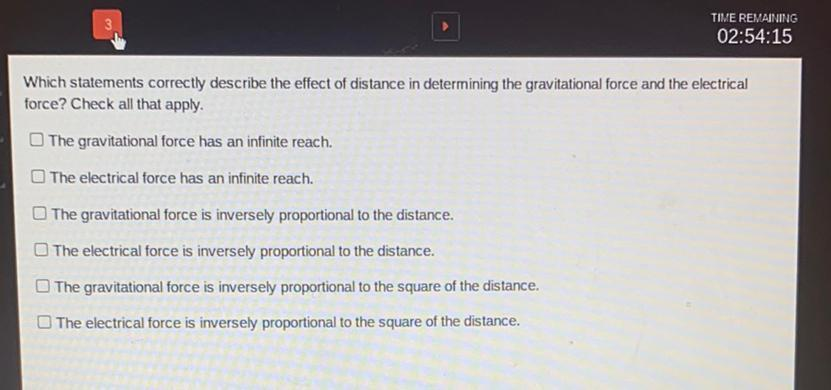Question:

# Which statements correctly describe the effect of distance in determining the gravitational force and the electrical force? Check all that apply. 1. The gravitational force has an infinite reach. 2. TWhich statements correctly describe the effect of distance in determining the gravitational force and the electrical force? Check all that apply. 1. The gravitational force has an infinite reach. 2. The electrical force has an infinite reach. 3. The gravitational force is inversely proportional to the distance. 4. The electrical force is inversely proportional to the distance. 5. The gravitational force is inversely proportional to the square of the distance. 6. The electrical force is inversely proportional to the square of the distance.